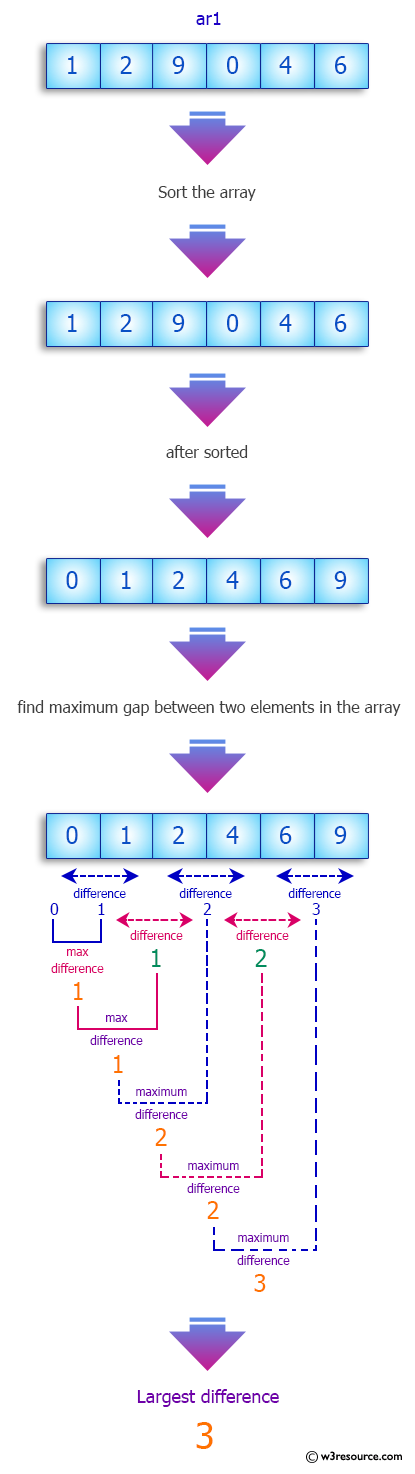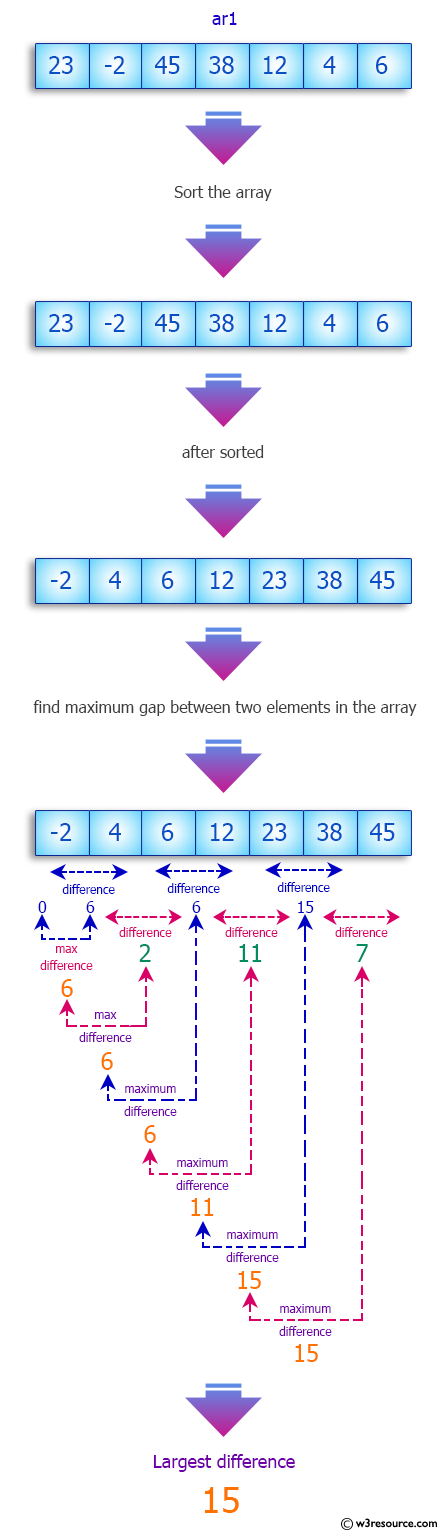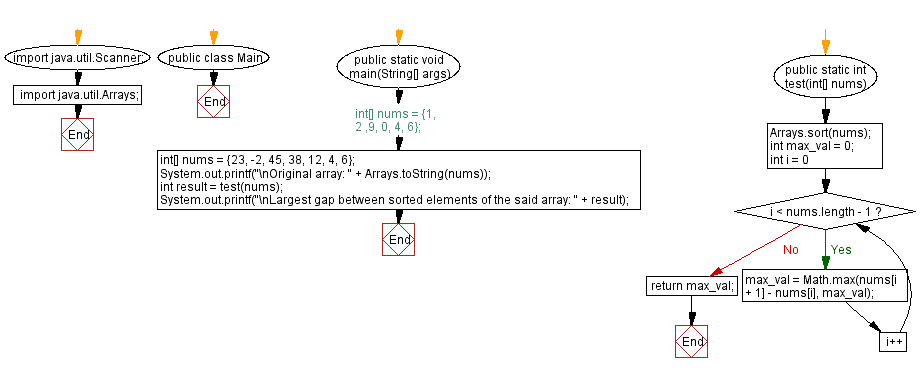﻿ Java -Largest gap between sorted elements of an array

# Java: Largest gap between sorted elements of an array

## Java Array: Exercise-75 with Solution

Write a Java program to calculate the largest gap between sorted elements of an array of integers.

Sample Data:
{1, 2 ,9, 0, 4, 6} -> 3
{23, -2, 45, 38, 12, 4, 6} -> 15

Pictorial Presentation:Sample Solution:

Java Code:

``````// Import necessary Java classes.
import java.util.Scanner;
import java.util.Arrays;

// Define the 'Main' class.
public class Main {
// Define the main method for running the program.
public static void main(String[] args) {
// Initialize an array of numbers.
//int[] nums = {1, 2 ,9, 0, 4, 6};
int[] nums = {23, -2, 45, 38, 12, 4, 6};
System.out.printf("\nOriginal array: " + Arrays.toString(nums));

// Call the 'test' method to find the largest gap between sorted elements of the array.
int result = test(nums);
System.out.printf("\nLargest gap between sorted elements of the said array: " + result);
}

// Define the 'test' method to find the largest gap between sorted elements of the array.
public static int test(int[] nums) {
// Sort the 'nums' array in ascending order.
Arrays.sort(nums);
int max_val = 0;

// Iterate through the sorted array and find the maximum gap between adjacent elements.
for (int i = 0; i < nums.length - 1; i++) {
max_val = Math.max(nums[i + 1] - nums[i], max_val);
}
return max_val;
}
}
```
```

Sample Output:

```Original array: [23, -2, 45, 38, 12, 4, 6]
Largest gap between sorted elements of the said array: 15
```

Flowchart:Java Code Editor:

Previous Java Exercise: Find all triplets equal to a sum in a unsorted array.
Next Java Exercise: Consecutive Numbers in an array.

What is the difficulty level of this exercise?

Test your Programming skills with w3resource's quiz.

﻿